# Valuing companies with Excel & Python — Part 2 : Historical Assessment and Model Building

“All models are wrong, but some are useful” – George Box

# 1. Context is Key — Historical Assessment

`#visual inspection of assumptionsws = wb.sheets['3 Statement Page']d = {}for row in it.chain(range(5,16), range(18,25),range(28,39), range(41,45), range(48,54),range(56,61), range(63,67),range(69,72)):    rw = []    for col in range(5,20):        char = get_column_letter(col)        rw.append(ws[char + str(row)].value)    d[ws['C'+str(row)].value] = rwdf = pd.DataFrame(d).transpose()        df.columns = group.loc['fillingDate'][::-1][-15:]df.loc['fillingDate'] = pd.to_datetime(group.loc['fillingDate'][::-1][-15:]).valuesfor i in range(len(df)-1):    ts = pd.DataFrame([df.loc[list(d.keys())[i]], df.loc['fillingDate']]).transpose().dropna()    ts = ts.set_index('fillingDate')                   fig, ax = plt.subplots()    ax.plot(ts)    ax.set_ylabel(list(d.keys())[i])    plt.title(f'{ticker} {list(d.keys())[i]}')`
1. Gross or Operating Margin trend — is the margin improving or worsening over time?
2. D&A vs Capital Expenditure — is the business under or over depreciating its assets?
3. Cash Conversion Cycle — is the business getting more or less favourable terms against its customers and suppliers?
4. Goodwill — is the company acquiring to grow, and is it possibly overpaying?
5. Debt — what kind of debt is the company using? Is it increasing or decreasing leverage? What terms is it getting on that debt?
6. Cash flows — are operating cash flows growing? Is the business investing consistently? Is it a net payer or receiver of financing?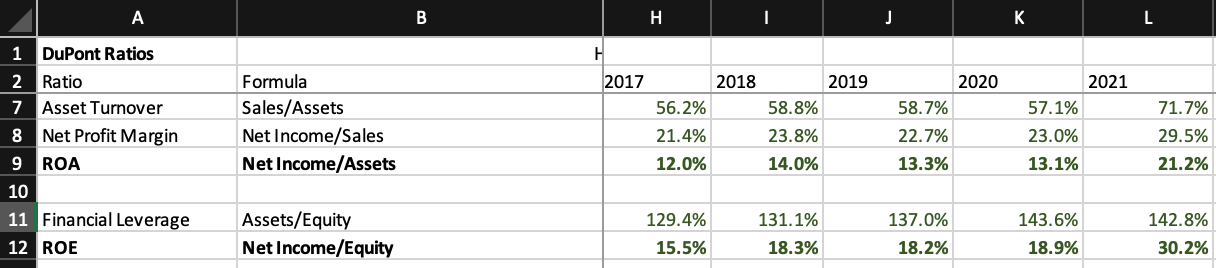DuPont Analysis of Alphabet since 2017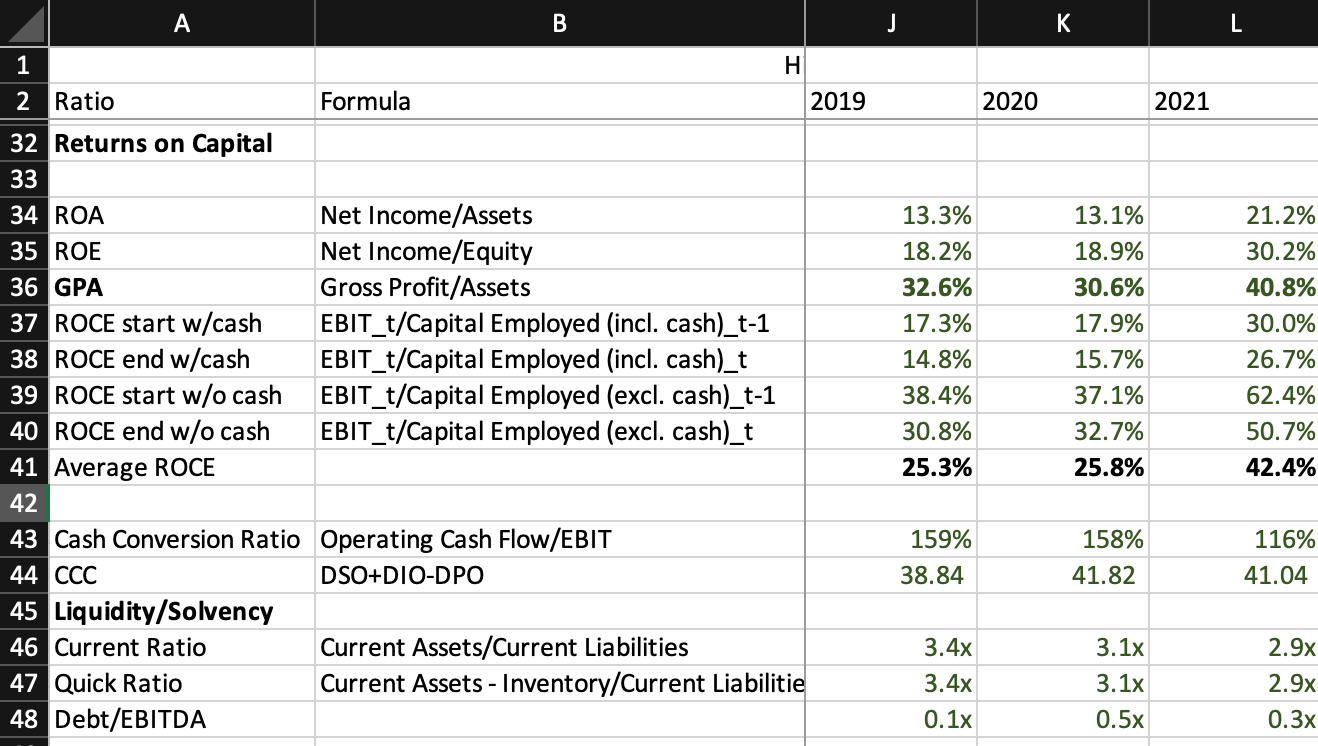Returns on Capital Metrics, Cash Conversion Metrics and Liquidity Metrics

# 2. Looking Through a Fogged Windshield — Modelling the Financial Statements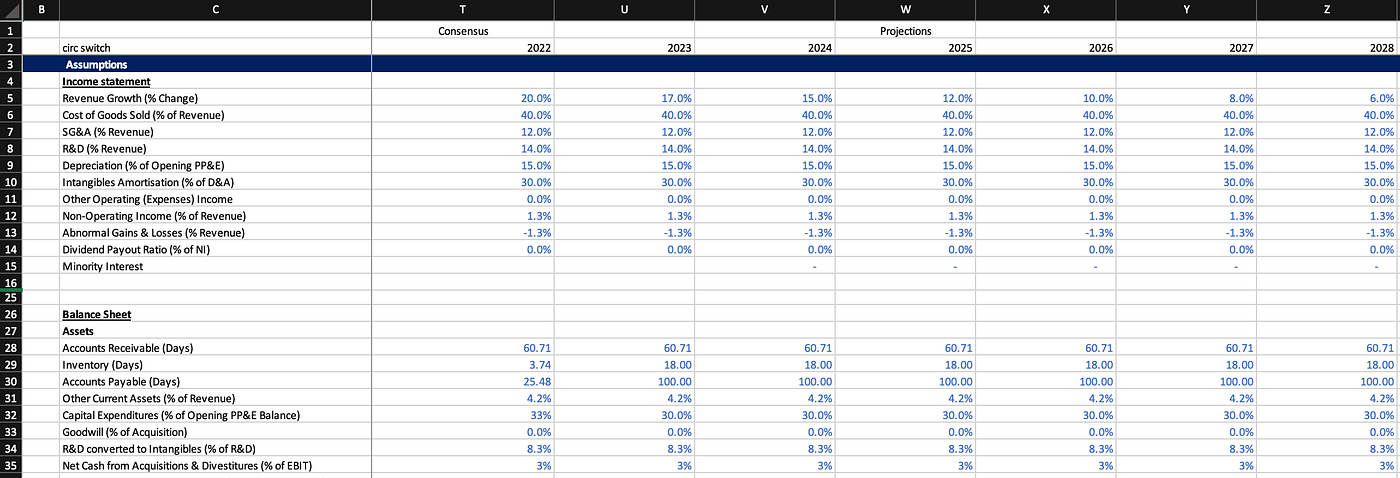Modelling Income Statement and Balance Sheet Assumptions
`lb_five = df.iloc[:,-5:].mean(axis = 1, skipna = True)lb_five_to_ten = df.iloc[:,-10:-5].mean(axis = 1, skipna = True)lb_ten_to_fifteen = df.iloc[:,-15:-10].mean(axis = 1, skipna = True)alpha = 0.4ema_estimates = alpha * lb_five + (1 - alpha) * (alpha * lb_five_to_ten + (1 - alpha) * lb_ten_to_fifteen)variance = df.std(axis = 1, skipna = True)`
`revenue_growth  =    [0.20,0.17,0.15,0.12,0.10,0.08,0.06]cost_of_goods   =    [0.4,0.4,0.4,0.4,0.4,0.4,0.4]sg_a            =    [0.12,0.12,0.12,0.12,0.12,0.12,0.12]r_d_expense     =    [0.14,0.14,0.14,0.14,0.14,0.14,0.14]d_a_rate        =    [0.15,0.15,0.15,0.15,0.15,0.15,0.15]amort_rate      =    [0.3,0.3,0.3,0.3,0.3,0.3,0.3,0.3]beta            =    [0.95]exit_multiple   =    end_growth      =    [0.02]ws['T5:Z5'].value = revenue_growthws['T6:Z6'].value = cost_of_goodsws['T7:Z7'].value = sg_aws['T8:Z8'].value = r_d_expensews['T9:Z9'].value = d_a_ratews['T10:Z10'].value = amort_ratews['R309'].value = betaws['V306'].value = exit_multiplews['V308'].value = end_growthbase_valuation_em = ws.range('S347').valuebase_valuation_ggm = ws.range('T347').value`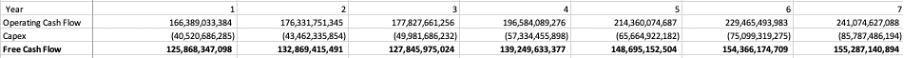Projected Free Cash Flow for Alphabet 2022–2028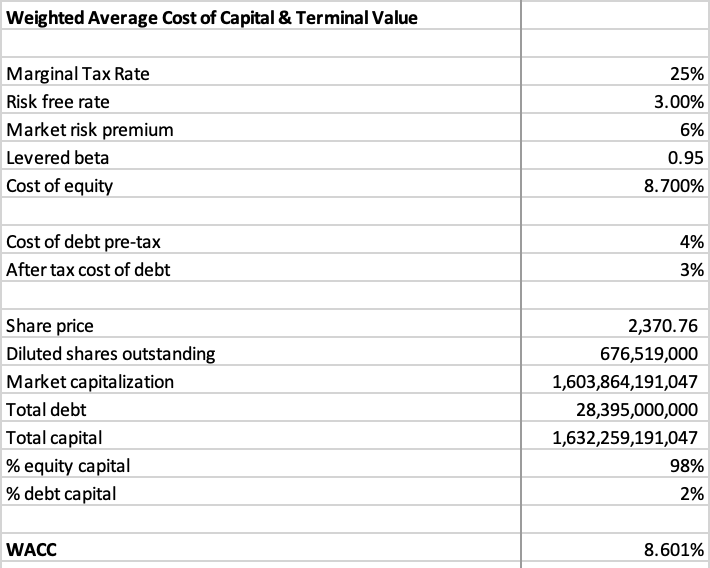Weighted Average Cost of Capital Calculation for Alphabet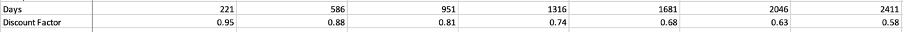Discount Factors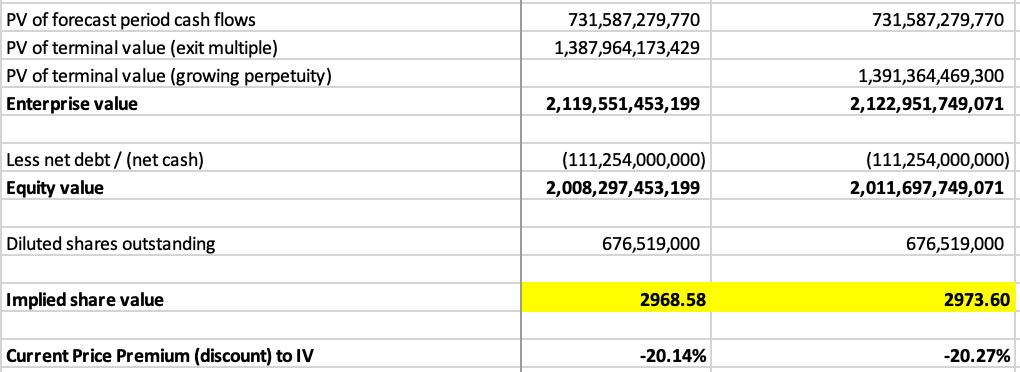Base Case Valuation of Alphabet Using an EV/EBITDA and GGM Approach